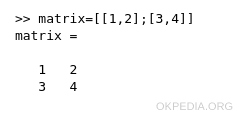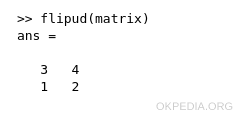# How to flip a matrix vertically in Matlab and Octave

To flip a matrix vertically in Matlab and Octave use the function flipud.

flipud(m)

The m parameter is the name of the matrix to flip.

Example

Define a matrix 2x2

matrix=[[1,2];[3,4]]

It is a square matrix.The first row contains the elements 1 and 2.

The second row contains the elements 3 and 4.

Flip the matrix vertically using the function flipud()

flipud(matrix)

The output result is a new matrixThe first row of the matrix became the second row. And viceversa.

https://how.okpedia.org/en/matlab/how-to-flip-a-matrix-in-matlab-and-octaveReport us an error or send a suggestion to improve this page

Matrix in Matlab/Octave